# Maths - Cubical Type Theory (CTT)

Cubical type theory (CTT) has some nice properties if we want to represent mathematical structures in a computer and do constructive proofs (which I do).

 On the page here Martin-Löf type theories are introduced and here is a discussion of how it can be used in the Idris language. The aim of this page is to combine these ideas with:### Motivation

 This motivation is very hand-wavy, it is not intended to be rigorous, its just my attempt to get some intuition. To prove weaker forms of equality such as isomorphism we usually have two functions between the objects and require some equations to be true. This is an external, category theory, type of approach which requires some functions/arrows to exist but doesn't say exactly what they are. What we want here is to internalise it and make it more constructive.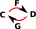One approach might be just have a single arrow in one direction, this is possible and allows us to define weak equivalence This arrow defines how homsets are mapped. This has its limitations and we have to arbitrarily decide the direction of the arrow.What we do here is to have a 'function' into this line between the 'equal' objects. This line comes from an interval.### Paths

In this theory equalities are represented by a path, in a given space, between equal terms.

CTT involves some indirection, so a path is a 'map' from an interval (denoted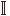) to the proposition that we are proving.

 A path can be defined like a function out of the interval p:-> A However, when denoted like that, the endpoints are not visible outside the function (at compile time) so its better to internalise it (with the endpoints as parameters) so the endpoints are visible and denote it like this: PathAx y where: p 0 = x p 1 = y Althoughis not strictly a type so we have to be careful how we work with it, we can't have a function into it for example.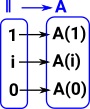Such a path 'type' is a proposition that x and y are equal. We can have such 'types' regardless of whether they are true or false. To prove that this is a true proposition we have to construct an instance of it.

 A map out of an interval is not strictly a function, if it was a function it could be constructed with a lambda function like this: p=λi.expression(i) but it is still a binding of the variable 'i' so instead we write it like this: p=expression(i)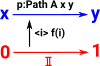So the Path construct is like a function type but is not strictly a type so we can summarise the different notation as follows:

Function

Path

function out of Interval

Interval
formation A->B Path A a b
constructor λ x.exp(x) p=<i>exp(i)
eliminator f(x) p@i

### Interval

 An interval spaceis a space with two points and a path between them. The interval is like a type, we think of functions from it. Technically it is not exactly a type, for instance, we can't have functions into it. 0 -> 1 We can have a variable (denoted 'i' here) to range between these two values.If there are more variables then there are also meets and joins.## Example n=n+0

 To represent an equality like this we use a path. In the language cubicaltt, when a path is used in a type like Nat directly (heterogeneous path type) it is denoted by PathP. refl : PathP Nat n n+0
 For the reasons discussed on this page we instead work more indirectly in terms of a map out of the interval into Nat. This gives a more indirect notion of path denoted by Path in cubicaltt. When we have a function like x->y we interpret this as y for all x. So-> Nat gives a type for all values along the interval. The n -> at the start shows we want a path that is true for all numbers. So we interpret the whole thing as an equality between the interpretation of n and the interpretation of n+0.If we want to prove n=n+0 for all n we want to prove, 0=0+0 1=1+0 2=2+0 and so on but since Nat is infinite we can never do it in this way. However Nat is defined inductively so we can prove this using induction.## Presheaf

### Presheaf Categories

A 'presheaf' category is a special case of a functor category (see page here). It is a contravarient functor from a category 'C' to Set.

Since it is contravarient Cop->Set the homset representing all possible functors can be written:

Hom(Cop,Set)

or

SetCop

There is more about presheaves on the page here.

### Cubical sets as presheaf

 We can think of this hom functor Cubeop -> set as picking out all cases of a shape (in this case cubes) in set.Cubical sets are presheaf categories (quote from site here):

We need cubical because products are closed. This gives 'tiny' interval II in the presheaf category.

For a discussion about the sheaf stucture of simplicial sets see the page here.

### Presheaf Example - Graph### Presheaf - IntervalThis diagram tries to relate this to cubical complex bases on combinatorial notation as described on page here.A slightly more complex complex:### Kan Extensions

The concept of Kan extensions comes from category theory and is introduced on the page here.

 We are given 3 categories 'C', 'D' and 'E' and 2 functors F:C->E and K:C->D. The Kan extension tries to find some unique functors between D and E:
 Here we want to use this concept in cubical type theory: So, using the left Kan extension, C becomes the interval, D and E become types we want to compare.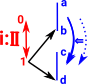According to this theory it suffices to define this at one end of the interval (say at i=1). The other end of the interval can be inferred.
 We can extend this concept to higher dimensional intervals (for example a square). Provided only one face is missing the orther one can be filled in See fill function on this site. In order to program with these concepts we may need the ability to work with individual arrows (partial functions). Extending one end extends the other.### Interval Eliminator

The eliminator for functions is function application which is usually indicated by juxtaposition. With a function out of the interval we use '@' to indicate function application. So, if we have a path from x to y we can use this to give us the endpoints.

• p @ 0 = x
• p @ 1 = y
 Example from Arend Language The function coe defines dependent eliminator for I, it says that in order to define f : \Pi (i : I) -> P i for some P : I -> \Type it is enough to specify f left ```\func coe (P : I -> \Type) (a : P left) (i : I) : P i \elim i | left => a``` From Arend code

### Consequences of Identity Eliminator

From the identity eliminator we can derive equivalence (symmetry, reflexitivity and transitivity) also substitution and unification.

#### Symmetry of Path

sym (A:U) (a b : A) (p:Path A a b) : Path A b a = <i>p e -1

#### Reflexitivity of Path

<i>a : Path A a a

a->a

<i>a can be thought of as like λ(i:)->a

That is constantly a.

#### Transitivity of Path

For every type A and every x,y,z there is a function:

(x=y) -> (y=z) -> (x=z)

#### Composition

 We want to compose two paths: Path A x y Path A y z These correspond to the bottom and right arrows on this diagram: Their composition is the arrow on the top.#### Unification

The process of finding a substitution that makes two atomic sentences identical.

#### Meet

Here we have two variables i and j. We want to look at p at i/\j which is given by:

<i,j>p@(i/\j)

by substituting 0 and 1 into various combinations of i and j we can get expressions for the edges and points on the diagram:#### Join

In this case we look at p at i\/j which is given by:

<i,j>p@(i\/j)

and again we substitute 0 and 1 into various combinations of i and j:##### Example Bool

For instance in Bool, if we want to prove not(not(x))=x. We don't just have a point not(not(x)) and a point x and a line between them. Instead we have a path as described above.

 This path represents a proposition. To prove the proposition we need to construct the type. As this type is a function that is an implementation of the function.##### Example Set
 p: Path Set a b is a proposition in set. We can make a proposition of equality between any two elements. <.> x:p is a constructor for this proposition, an implementation of the function out of the interval, so it is a proof of the proposition. In set we can only construct Path Set a a , so this is like Refl for set p: Path Set a b <.> x:p
##### Paths and Equivalence

Transport turns path into equivalence.

### Product Type

 Instances of the product type are a tuple. If we require (a,b)=(c,d) then we need a=c and b=d. (eliminator for product type).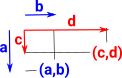### Topological Spaces As Types

 As discussed on the page here HoTT relates types to spaces: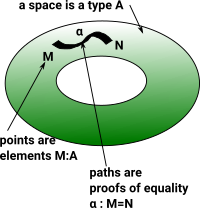On the page here is a discussion of proofs and how points can be considered equal if they have the same properties.There is a problem with modeling spaces in this way in the consistency of higher order equalities. To maintain consistency we need to model in a a less direct way by using the map out of an interval.### Definition

Every type needs to define

• composition operators: hcomp
• coertion operators: transp

### Comparison to Proof in Martin-Löf Theory

 On the page here we looked at proofs in a more traditional Martin-Löf theory. When implemented (modeled) in Idris it is shown as an equality which we understand as a proposition. `(plus x Z) = x` To prove that this proposition is true we need to construct it like this: `Refl` In order to use cubical type theory as implemented (modeled) in 'cubicaltt' we need some changes. Instead of '=' it uses Path. `Path nat (add zero n) n` This path is constructed as a function from the interval like this: ` zero`
 So here is a proof in Idris using traditional Martin-Löf theory. ```total plusZeroRightNeutral : (x : Nat) -> (plus x Z) = x plusZeroRightNeutral Z = Refl plusZeroRightNeutral (S n) = rewrite (plusZeroRightNeutral n) in Refl``` Here is the same proof from 'cubicaltt'. Instead of '=' it uses Path. ```add_zero : (n : nat) -> Path nat (add zero n) n = split zero -> zero suc n -> suc (add_zero n @ i)```
 Where: PathP is a heterogeneous path type and is a builtin keyword. Path is a homogeneous path type and is defined here as a map from the interval to 'a'. So, instead of modeling '=' directly, it is instead modeled indirectly as 'Path'. ```-- Identity types Path (A : U) (a0 a1 : A) : U = PathP ( A) a0 a1 refl (A : U) (a : A) : Path A a a = a```

The interval <i> behaves like a type in some ways but not in others. See below for further discusion of the interval where it will be denoted as.

The first way of modeling proofs assumes that things can only be equal in one way but here we change this so that things can be equal in multiple different ways. The above example is comparing values so they can only be equal in one way. Below we compare bool to bool, this can be equal to itself in 2 ways:

• where true=true and false=false
• or where true=false and false=true
 Here (from this site) the second type of equality is constructed . Transporting true along this equality gives false and false gives true. isoToEquiv : type of A, type of B, A->B,B->A,y->path B,x->path A notK is a proof that not not b = b ```notEquiv : equiv bool bool = (not,isoToEquiv bool bool not not notK notK)``` We can glue these equalities together. ```-- Construct an equality in the universe using Glue notEq : Path U bool bool = Glue bool [ (i = 0) -> (bool,notEquiv) , (i = 1) -> (bool,idEquiv bool) ]```
 There are various related mathematical structures that give us multiple perspectives on this.### Contractible Space

A contractible space is one that can be continuously shrunk to a point within that space.

topological space X is contractible if:

• the identity map on X is null-homotopic,
• if it is homotopic to some constant map.

the following are all equivalent:

• X is contractible (i.e. the identity map is null-homotopic).
• X is homotopy equivalent to a one-point space.
• X deformation retracts onto a point. (However, there exist contractible spaces which do not strongly deformation retract to a point.)
• For any space Y, any two maps f,g: Y -> X are homotopic.
• For any space Y, any map f: Y -> X is null-homotopic.
 (from this site) ```-- contractible type isContr (A : U) : U = (x : A) * ((y : A) -> Path A x y) -- The fiber/preimage of a map: fiber (A B : U) (f : A -> B) (y : B) : U = (x : A) * Path B y (f x) -- A map is an equivalence if its fibers are contractible isEquiv (A B : U) (f : A -> B) : U = (y : B) -> isContr (fiber A B f y)```

### Variables

When using 'logic' notation we often specify variables in a context to the left of a 'turnstyle' symbol.
 Γ,n:Nat(n=n+0)
In programming languages we might specify variables separately somewhere in scope.
```Nat n
n -> (n = n+0)```

When using cubical type theory we can specify variables in the formal interval:

If 'C' is the context, containing the variables, then Cop is the formal interval and a path is a presheaf Cop->Set.

```-- Identity types

Path (A : U) (a0 a1 : A) : U = PathP (<i> A) a0 a1

refl (A : U) (a : A) : Path A a a =<i> a```

### The Formal Interval

HoTT uses an idea from topology, the interval.represents the unit interval [0,1]

not totally ordered like classic interval.is like a type but it is not a type. Instead a path is a primitive notion in HoTT.

 used for variables i... with grammar r,s ::= 0 | 1 | i | 1-r | r/\s | r\/s where: 0 is least element 0:(1->) 1 is greatest element 1:(1->) r,s are variables r/\s is meet representing min /\:(->->) r\/s is join representing max \/:(->->) 1-r is an involution (its own inverse) where: 1-0=1 1-1=0 1-(r\/s) = (1-r)/\(1-s) 1-(r/\s) = (1-r)\/(1-s) (1-r):(->)## Glue

Glue types give us univalance.

Glue types turn an equivalence of types into a path:

ua: (A B : U) -> A ~ B -> PathU A B

#### Theory

 If we have a type B and a partial type A that is equivalent to B where it is defined then we can glue them together to form a new type.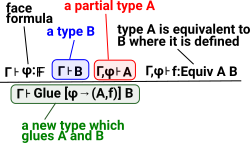#### Example

 Here is an example of a binary type with two elements '0' and '1'. This type can be 'equal' to inself in two ways: 0=0 and 1=1 or, 0=1 and 1=0So we get two squares that must commute.

Gluing the top mappings together then give one square and the centre of the square can be filled in representing the isomorphism/equality of the whole type with itself.

 Glue type from Agda cubical here ```Glue :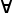{L L'} (A : Set L) {φ : I} -> Partial φ (Σ[TSet L'] TA) -> Set L'``` constructor ```glue :{ L L'} {A : Set L} {φ : I} {Te : Partial φ ( Σ[TSet L'] TA)} -> PartialP φ T -> A -> Glue A Te``` eliminator ```unglue :{ L L'} {A : Set L} (φ : I) {Te : Partial φ (Σ[TSet L' ] TA)} -> Glue A Te -> A``` Where: Partial - allows Agda to define partially specified n-dimensional cubes. Setω - allows Agda to make sure everything has a 'type' in the presence of universe polymorphism. `Partial :{L} -> I -> Set L -> Setω`

in agda

### Composition of Paths and Transport

These may need to be defined for each type. This is done using the glue construct above.

 For traditional equalities and equivalences we have a relatively simple concept of transitivity to combine them. So in the case illustrated here: A=B B=C so we can deduce A=CThis could be extended to multiple equalities which might be illustrated by a diagram like this where the missing face of a polygon can be inferred. This is related to a Horn clause discussed on page here.However, when we move to homotopy type equalities things get more complicated, we need to make sure all possible paths between the endpoints are included. See the discussion of composition of paths on page here.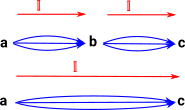### Example - List

 We have already looks at the 'bool' example. Here is slightly more complicated so that we can think about composition. So imagine a type which is a list containg the elements: 'x', 'y' and 'z'. If we have another list with these elements, but in a different order, then this would be isomorphic (but not equal) to the original list.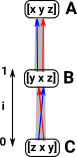### Combining intervals

 MeetJoin### Modeling Algebra

 I'm trying to put this all together in one diagram - not correct yet.### Comparison ETT and CTT

ETT CTT
Introduction

a,b:Nat

a=b

Path Nat a b
Construct Refl : a=b f:a->b
<i>f : Path Nat a b
Deconstruct

### Comparison ITT and HoTT

In M-L intentional type theory a proposition is either proven or not, if it was not then it may, or may not, be true. Since proofs in HoTT are canonical then we have other possibilities, it may be true, false, neither or both, also it may be true in many ways. These values represent truth values of propositions which correspond to paths in a space. This situation with different truth values is related to the idea of topos in category theory.

ITT HoTT

Proposition

a=b

Path

I->A
may still use a=b

Proof of that proposition

Refl: a=a

λi -> x

### Free De Morgan Algebra

De Morgan algebra is a generalisation of boolean algebra without rule of excluded middle.

#### Recap

So if we have 2 points 'n' and 'n+0' are they on a path/surface? This corresponds to the proposition 'n=n+0'. If we we using Boolean logic this proposition would be either true or false. In M-L type theory/constructive logic the proposition is either proven or its not, if its not then we can't say anything about it. Here the truth value is a free De Morgan algebra.### Fibrations

 A cubical complex might be thought of as a family of types where the cells are put into are hierarchy of types according to their dimension. These dimensions (and therefore types) are related by their boundary maps,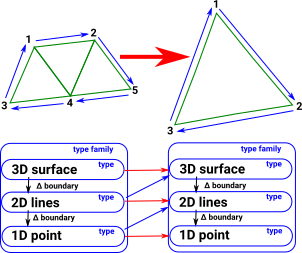### Presheaves

Presheaves allow us to build a more complex object out of simpler objects.

If C is a category of primitive objects then the presheaf is:

F: Cop -> Set

Objects of C Morphism in C
X
f: X -> Y
where:
F(X) is a set representing the ways X occurs inside F.

where:
F(f) : F(Y) -> F(X) is the function mapping each occurrence y of Y in F to the corresponding suboccurrence x (included in y through f) of X in F

 see nlab siteCubical sets are presheaves

Sheaves are discussed on page here.

## Implementations

 Agda CubicalTT Arend

Where I can, I have put links to Amazon for books that are relevant to the subject, click on the appropriate country flag to get more details of the book or to buy it from them.Computation and Reasoning - This book is about type theory. Although it is very technical it is aimed at computer scientists, so it has more discussion than a book aimed at pure mathematicians. It is especially useful for the coverage of dependant types.